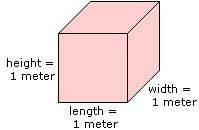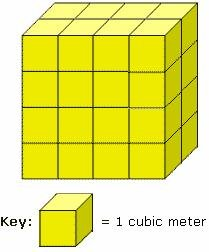## Definition Of Cubic Meter

Cubic Meter is a standard metric unit for measuring volume.

1 cubic meter = 1,000 liters
1 liter = 0.001 m3
A cubic meter can be abbreviated as m3 or cu m.

### Example of Cubic MeterThe volume of the cube i.e. V = length × width × height
= 1 meter × 1 meter × 1 meter
= 1 cubic meter.

### Solved Example on Cubic Meter

#### Ques: Find the volume of the solid.##### Choices:

A. 27 cubic meters
B. 16 cubic meters
C. 36 cubic meters
D. 32 cubic meters